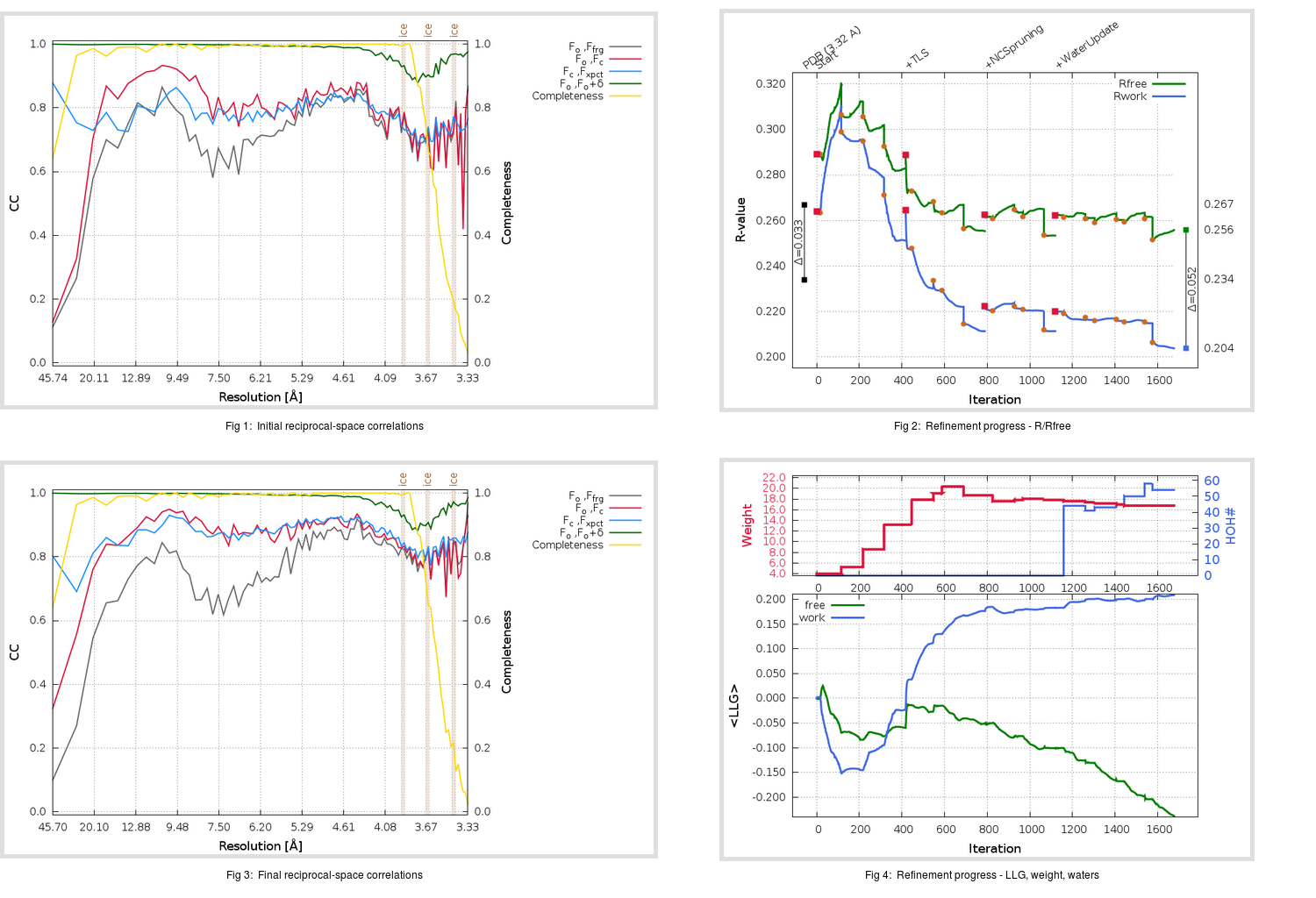Content:

```    Diffraction limits & principal axes of ellipsoid fitted to diffraction cut-off surface:
3.433         1.0000   0.0000   0.0000       a*
3.742         0.0000   1.0000   0.0000       b*
3.264         0.0000   0.0000   1.0000       c*
```

## Deposited

` `
 Date deposited Date data collection Resolution R, Rfree 20180119 20151210 3.32 0.2320 0.2670

Molprobity (CCP4 7.0 version) summary:

```Ramachandran outliers =   0.72 %
favored =  93.93 %
Rotamer outliers      =   8.48 %
C-beta deviations     =     0
Clashscore            =   8.99
RMS(bonds)            =   0.0037
RMS(angles)           =   0.99
MolProbity score      =   2.59
Resolution            =   3.32
R-work                =   0.2320
R-free                =   0.2670
```

```Number of waters      =     0

<B> (all atoms) =   87.25 ( sd =   48.29 ) for       9703 non-hydrogen atoms
<B>   (protein) =   87.24 ( sd =   48.29 ) for       9698 non-hydrogen atoms
<B>     (water) =    0.00 ( sd =    0.00 ) for          0 non-hydrogen atoms
<B>    (others) =  116.21 ( sd =    0.45 ) for          5 non-hydrogen atoms

B min/max       (all non-hydrogen atoms) =    4.17 /  397.97
B min/max   (protein non-hydrogen atoms) =    4.17 /  397.97
B min/max     (water non-hydrogen atoms) =    0.00 /    0.00
B min/max     (other non-hydrogen atoms) =  115.49 /  116.81
```

## BUSTER (re-)refinement

` `

Molprobity (CCP4 7.0 version) summary:

```Ramachandran outliers =   2.16 %
favored =  90.89 %
Rotamer outliers      =  13.25 %
C-beta deviations     =    11
Clashscore            =   8.15
RMS(bonds)            =   0.0120
RMS(angles)           =   1.70
MolProbity score      =   2.82
Resolution            =   3.32
R-work                =   0.2038
R-free                =   0.2556
```

```Number of waters      =    54

<B> (all atoms) =   89.04 ( sd =   30.92 ) for       9757 non-hydrogen atoms
<B>   (protein) =   89.35 ( sd =   30.68 ) for       9698 non-hydrogen atoms
<B>     (water) =   29.93 ( sd =   12.73 ) for         54 non-hydrogen atoms
<B>    (others) =  124.57 ( sd =    0.04 ) for          5 non-hydrogen atoms

B min/max       (all non-hydrogen atoms) =    6.86 /  193.28
B min/max   (protein non-hydrogen atoms) =   35.13 /  193.28
B min/max     (water non-hydrogen atoms) =    6.86 /   60.43
B min/max     (other non-hydrogen atoms) =  124.53 /  124.63
```

Refinement progression:Results:

` `
 File Remark 6C6Y_aB_refine.01_04_refine.pdb.gz exact refinement commands are in header 6C6Y_aB_refine.01_04_refine.mtz.gz including original deposited data and several re-refinement map coefficients 6C6Y_aB_refine.01_04_BUSTER_model.cif.gz including any non-standard compound restraints 6C6Y_aB_refine.01_04_BUSTER_refln.cif.gz